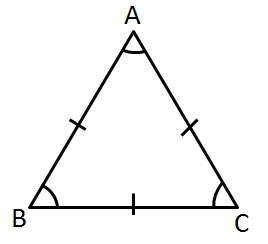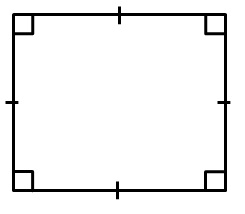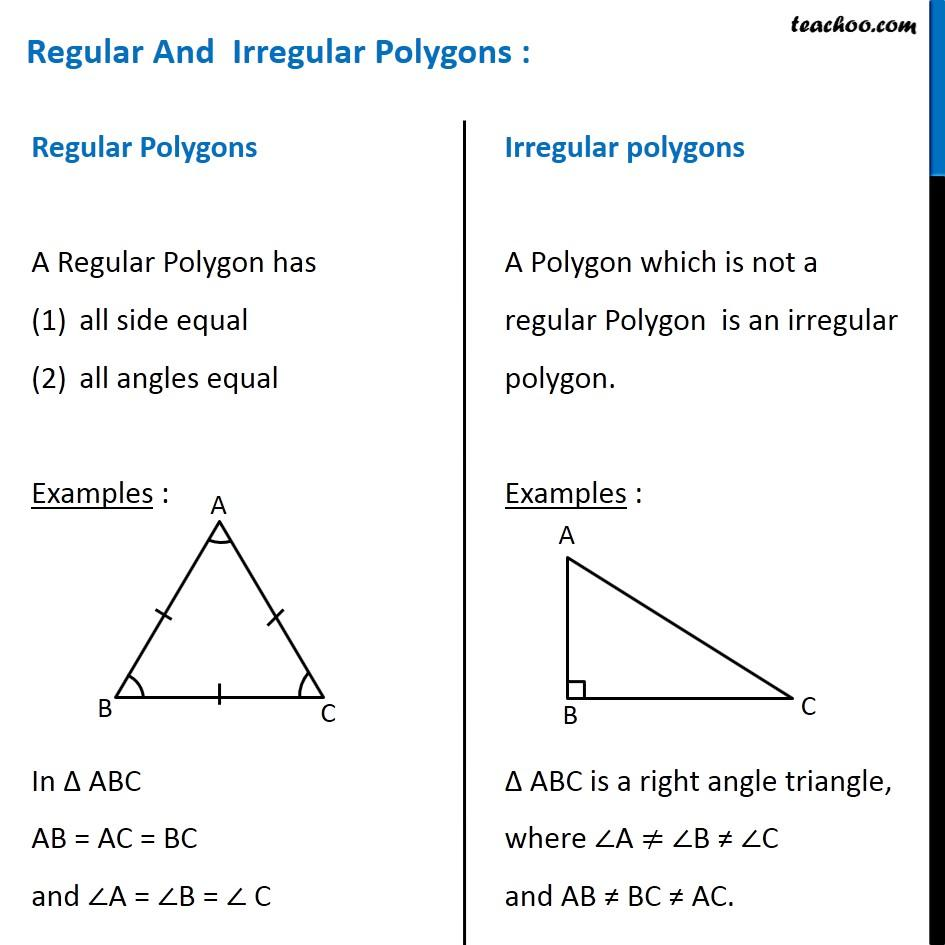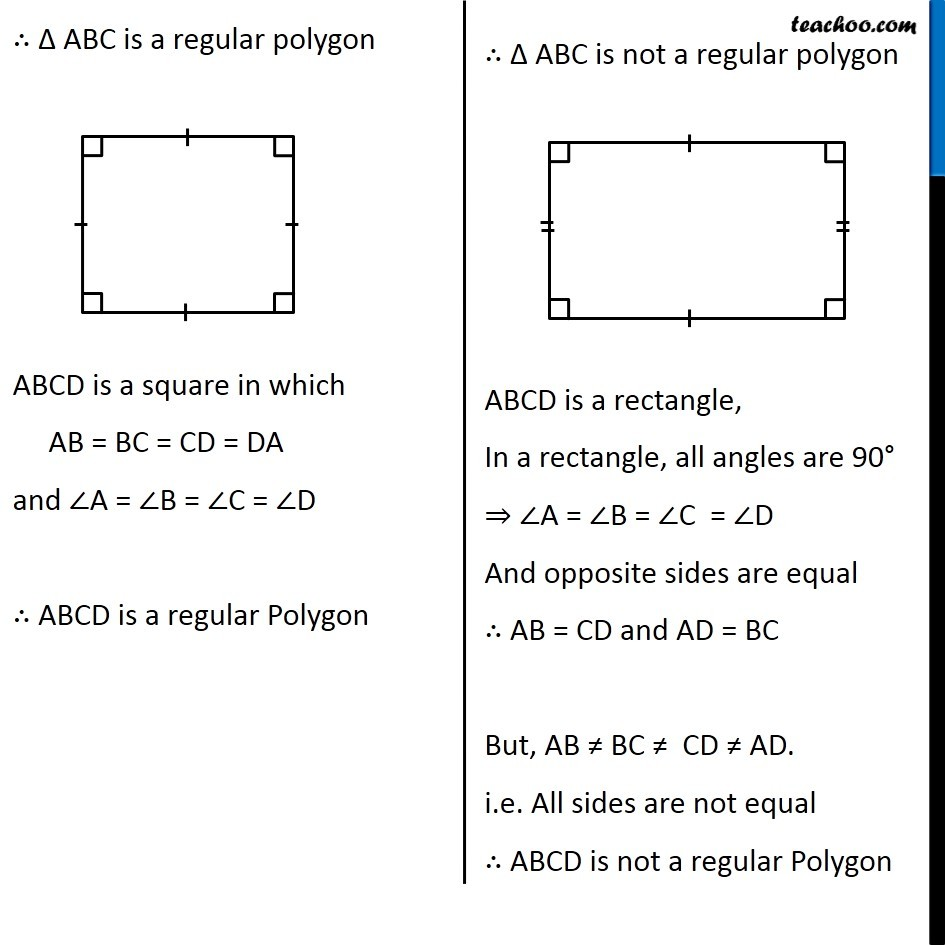Polygons

Chapter 3 Class 8 Understanding Quadrilaterals
Concept wise

Regular Polygons

A Regular Polygon has

(1) all side equal

(2) all angles equal

Examples :In ∆ ABC

AB = AC = BC

and ∠A = ∠B = ∠ C

∴ Δ ABC is a regular polygonABCD is a square in which

AB = BC = CD = DA

and ∠A = ∠B = ∠C = ∠D

∴ ABCD is a regular PolygonLearn in your speed, with individual attention - Teachoo Maths 1-on-1 Class

### Transcript

Regular Polygons A Regular Polygon has all side equal all angles equal Examples : In ∆ ABC AB = AC = BC and ∠A = ∠B = ∠ C Irregular polygons A Polygon which is not a regular Polygon is an irregular polygon. Examples : Δ ABC is a right angle triangle, where ∠A ≠ ∠B ≠ ∠C and AB ≠ BC ≠ AC. ∴ Δ ABC is a regular polygon ABCD is a square in which AB = BC = CD = DA and ∠A = ∠B = ∠C = ∠D ∴ ABCD is a regular Polygon ∴ Δ ABC is not a regular polygon ABCD is a rectangle, In a rectangle, all angles are 90° ⇒ ∠A = ∠B = ∠C = ∠D And opposite sides are equal ∴ AB = CD and AD = BC But, AB ≠ BC ≠ CD ≠ AD. i.e. All sides are not equal ∴ ABCD is not a regular Polygon